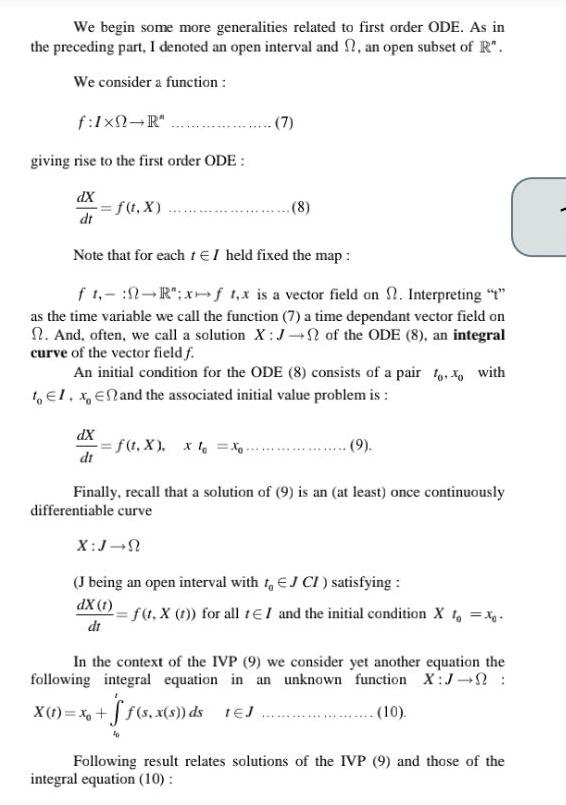Question:

# We begin some more generalities related to first order ODE

Last updated: 9/18/2023We begin some more generalities related to first order ODE As in the preceding part I denoted an open interval and an open subset of R We consider a function f 1x0 R giving rise to the first order ODE dX dt f t x dX dt 7 Note that for each t I held fixed the map ft R x f x is a vector field on Interpreting t as the time variable we call the function 7 a time dependant vector field on n And often we call a solution X J of the ODE 8 an integral curve of the vector field f An initial condition for the ODE 8 consists of a pair too with to El x En and the associated initial value problem is f t X x t Xo 8 9 Finally recall that a solution of 9 is an at least once continuously differentiable curve X J N J being an open interval with to EJ CI satisfying dX 1 dt 2 f t X t for all t I and the initial condition X t xq In the context of the IVP 9 we consider yet another equation the following integral equation in an unknown function X J X 1 x ff s x s ds 1 J 10 Following result relates solutions of the IVP 9 and those of the integral equation 10